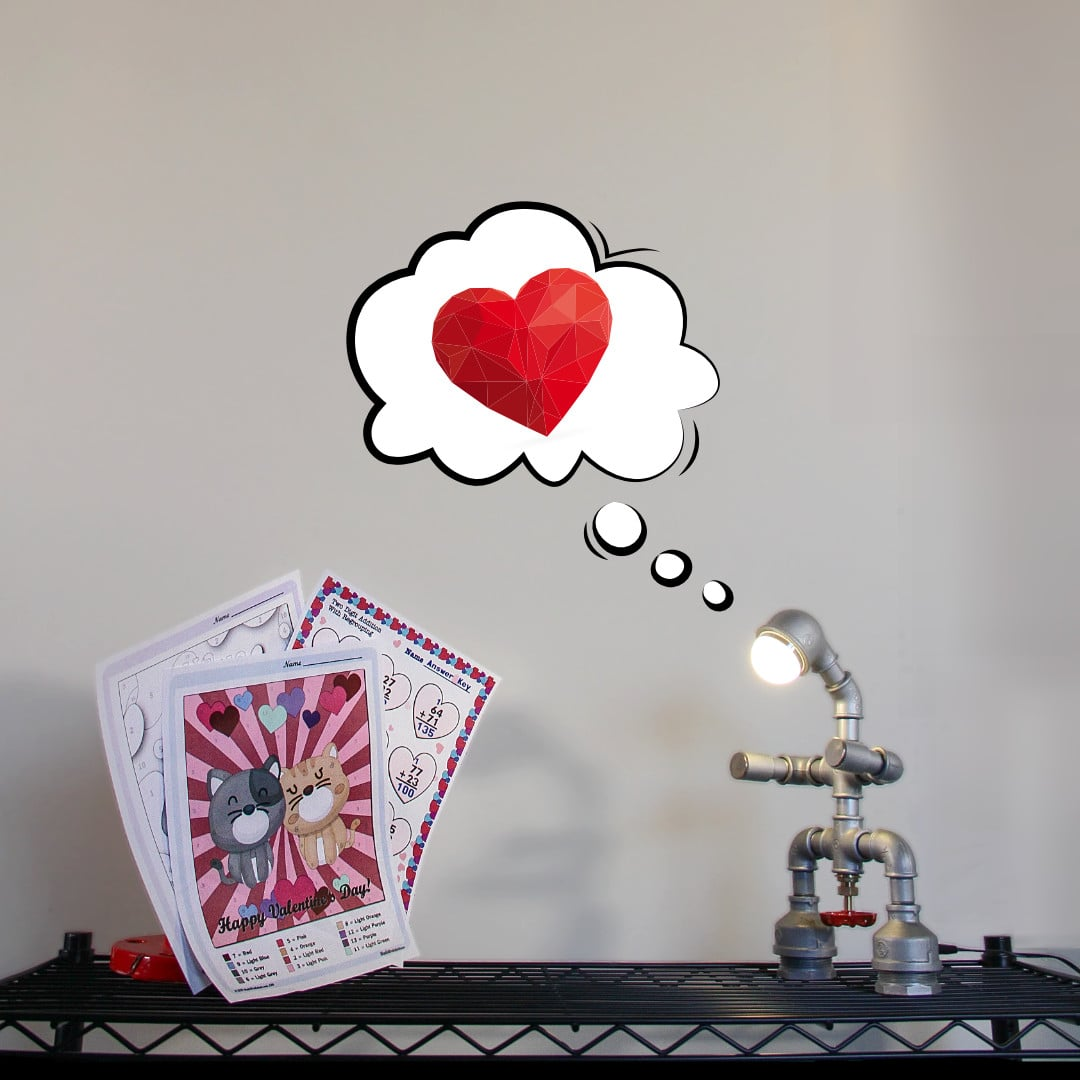# Valentine's Day

Looking for worksheets to make learning math on Valentine's Day a bit more fun? This page has a collection of color by number worksheets appropriate for kindergarten through fourth grade, covering addition, subtraction, multiplication and division operations. There are also a collection of simple math exercises with fun Valentine's Day themes.

## Get Some Valentine's Day Math Practice!

If you're celebrating Valentine's Day, it can be a lot of fun to introduce some math practice into the season!

The worksheets on this page feature both math fact practice and color by number activities, including coloring pages that incorporate math facts for addition, subtraction, multiplication and division. Students solve basic bath fact problems, and use the answers to determine what color to apply to different areas of the worksheet.

The theme for Valentine's Day is obviously romance, and the coloring worksheets feature a couple, penguins, giraffes and kittens (naturally!) to be revealed as grade school students color in their answers.

These worksheets are appropriate for students in kindergarten through 4th grade. The color by number pages are great for kindergarten, and some of the addition worksheets can be introduced at that grade level as well. The addition and subtraction worksheets are appropriate for first grade and second grade students, which the multiplication and division worksheets more appropriate for third and 4th grade students.